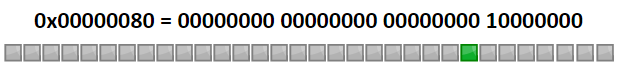# Type Conversion in Java

Type conversion means converting a variable of one data type to another.

For example, we can assign variable of integer data type into a variable of long data type. Now, this is just a simple example of type conversion. There are other types of conversion as well in Java.

### Java has two types of conversion:

1.    Automatic Conversion / Implicit conversion
2.    Explicit Conversion

## Automatic Conversion / Implicit conversion

Automatic conversion is the type of conversion where we don’t need to do any explicit conding for converting a datatype to another, we just assign a variable into another.

Consider the following example:

``````public class Conversion {

public static void main(String[] args) {
int i = 10;
long l = i;
System.out.println("The long value is : " + l);
}

}``````

Output :

The long value is : 10

What we can see the red box is , we simply assigned the integer value into the long value. But, can we just do this same thing to any and all the variables?.

For example, if we want to assign the integer into some byte variable, it will throw an error in compilation time

### So, there must be some rules for that!

Surely, it does. Automatic Conversion is possible if only the following properties are satisfied.

• The two types are compatible.
One data type is compatible to the another data type, if values of one type can appear wherever the values of other type is expected.
• The destination type is larger than the source type

“Destination type is larger than the source”  means – the size of the destination data type should be larger than the size of the source data type.

In the above example, size of int is 32 bits where size of long in 64 bits. So, we were able to assign a int value in a long.

At the same time, byte has a size of 8 bits, so when we try to assign a int value in a byte variable, it throws compilation error.

## Explicit Conversion

Sometimes we may need to convert a datatype into another where the source and the destination datatypes are not compatible.

For example, few minutes back we tried to convert a integer into a byte variable. For this kind of converisons we need explicit conversion.

But, keep in mind that in this kind of conversion there is a possiblilty of data loss.

### Syntax :

destination_variable = (destination_datatype) source_variable

Example – integer to byte

``````public class Conversion {

public static void main(String[] args) {

int i = 128;
byte b = (byte) i;
System.out.println("The byte value is : " + b);
}

}``````

The output will be :

The byte value is: 1

Why the byte is showing 1?

First convert integer 128 to byte :Size of byte is 8 bits. So, while converting inetegr to a byte, Java will take the right most 8 bits and convert I tto byte. Rightmost 8 digits are: 100000000

Now, we know that Byte is a signed data type whose leftmost digit is for sign. Thus 10000000 will be (128-256) = -128 in Byte. And, -128 is the result.

Example – double to byte

``````public class Conversion {

public static void main(String[] args) {

double d = 128.45;
byte b = (byte) d;
System.out.println("The byte value is : " + b);
}

}``````

Output :

The byte value is : -128

In the above program, we are trying to assign a double value to a byte. It works slightly differently.

For decimal number, first they are converted to inetgers and then the rest of the process in same as process for converting from integers to bytes.

For the above example:

double d = 128.45;

After converting it to integer, it will compile become 128.

Now we will convert it to byte variable, in the same way as mentuoned above. The result will be -128.

The output of the program is :

The byte value is : -128

## Automatic Type Promotion

While evaluating an expression of byte, short or char, Java automatically promotes the datatype of the variables to int.

Example :

``````public class Conversion {

public static void main(String[] args) {
byte b1 = 3;
byte b2 = 4;
int i = b1 * b2;
System.out.println("The multiplication result is : "+ i);
}

}``````

Output:

The multiplication result is : 12

In this code, we didn’t assigned bytes to integers. While multiplications, it automaticaly gets promoted to integer.

Rules of Type Promotion

1.    Byte, short and characters will ebe promoted to integer while evaluation of the expressions.

2.    If any one of the operand in the expression is a long, the whole expression converts to long.

3.    If any one of the operands in the expression in float, the whole expression converts to float.

4.    If any one of the operands in the expression in double, the whole expression converts to double.

### Wideing and Narrowing Type Conversion

These two names are fancy names for the types conversions we learned above.

### Widening Type Conversion :

is the conversion where the destination datatype has a bigger size than the source datatype . So, implicit conversions comes under this category.

### Narrowing Type Conversion :

is the conversion where the destination datatype has a smaller size than the source datatype.
Thus, there is a possibility of data loss while type conversion. So, as we can understand, Explicit type conversion is of this type.

Scroll to Top